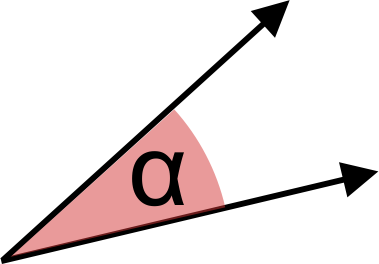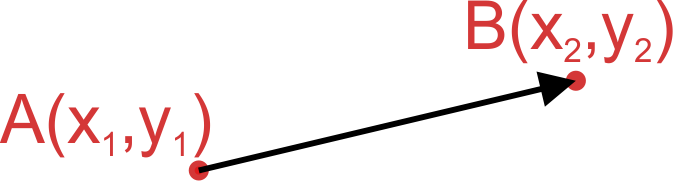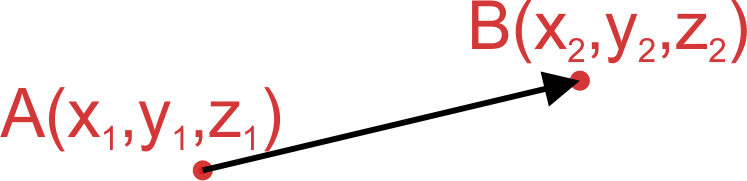# Angle Between Two Vectors Calculator

Created by Hanna Pamuła, PhD candidate
Reviewed by Bogna Szyk and Jack Bowater
Last updated: May 13, 2022

With this angle between two vectors calculator, you'll quickly learn how to find the angle between two vectors. It doesn't matter if your vectors are in 2D or 3D, nor if their representations are coordinates or initial and terminal points - our tool is a safe bet in every case. Play with the calculator and check the definitions and explanations below; if you're searching for the angle between two vectors formulas, you'll definitely find them there.

Since you're here, hunting down solutions to your vector problems, can we assume that you're also interested in vector operations? If you want to start from the basics, have a look at our unit vector calculator. For those who want to dig even more into vector algebra, we recommend the [vector projection direction of the vectortool](calc:2030) and the cross product calculator. Last but not least, if you only have one vector and want to find the angle it forms with the z-axis, take a look at our direction of the vector calculator.

## Angle between two vectors formulas

In this paragraph, you'll find the formulas for the angle between two vectors - and only the formulas. If you'd like to understand how we derive them, go directly into the next paragraph,Angle between two 2D vectors

1. Vectors represented by coordinates (standard ordered set notation, component form):vectors a = [xa, ya] , b = [xb, yb]

angle = arccos[(xa * xb + ya * yb) / (√(xa2 + ya2) * √(xb2 + yb2))]

1. Vectors between a starting and terminal point:For vector a: A = [x1, y1] , B = [x2, y2],

so vector a = [x2 - x1, y2 - y1]

For vector b: C = [x3, y3] , D = [x4, y4],

so vector b = [x4 - x3, y4 - y3]

Then insert the derived vector coordinates into the angle between two vectors formula for coordinate from point 1:

angle = arccos[((x2 - x1) * (x4 - x3) + (y2 - y1) * (y4 - y3)) / (√((x2 - x1)2 + (y2 - y1)2) * √((x4 - x3)2 + (y4 - y3)2))]

Angle between two 3D vectors

1. Vectors represented by coordinates:a = [xa, ya, za] , b = [xb, yb, zb]

angle = arccos[(xa * xb + ya * yb + za * zb) / (√(xa2 + ya2 + za2) * √(xb2 + yb2 + zb2))]

1. Vectors between a starting and terminal point:For vector a: A = [x1, y1, z1], B = [x2, y2, z2],

so a = [x2 - x1, y2 - y1, z2 - z1]

For vector b: C = [x3, y3, z3], D = [x4, y4, z4]

so b = [x4 - x3, y4 - y3, z4 - z3]

Find the final formula analogically to the 2D version:

angle = arccos{[(x2 - x1) * (x4 - x3) + (y2 - y1) * (y4 - y3) + (z2 - z1) * (z4 - z3)] / [√((x2 - x1)2 + (y2 - y1)2+ (z2 - z1)2) * √((x4 - x3)2 + (y4 - y3)2 + (z4 - z3)2)]}

Also, it is possible to have one angle defined by coordinates, and the other defined by a starting and terminal point, but we won't let that obscure this section even further. All that matters is that our angle between two vectors calculator has all possible combinations available to you.

## How to find the angle between two vectors?

OK, the above paragraph was a bit of a TL;DR. As a way of better understanding the formulas for the angle between two vectors, let's check where they come from:

The dot product is defined as the product of the vectors' magnitudes multiplied by the cosine of the angle between them (here denoted by α):

a · b = |a| * |b| * cos(α)

2. Then, make the angle the subject of the equation:

Divide by the product of the vectors' magnitudes:

cos(α) = a · b / (|a| * |b|)

Find the inverse cosine of both sides:

α = arccos[(a · b) / (|a| * |b|)]

3. Afterward, we need to brush up on the definition of a vectors' magnitude:

As magnitude is the square root of the sum of the vector's components to the second power, we find out that:

• |v| = √(x² + y²) in 2D space

• |v| = √(x² + y² + z²) in 3D space

Did you notice that it's the same formula as the one used in the distance calculator? And that it comes directly from geometry - that is, the Pythagorean theorem?

1. Use the algebraic formula for the dot product (the sum of products of the vectors' components), and substitute in the magnitudes:
• in 2D space

If vectors a = [xa, ya], b = [xb, yb], then:

α = arccos[(xa * xb + ya * yb) / (√(xa2 + ya2) * √(xb2 + yb2))]

• in 3D space

If vectors a = [xa, ya, za], b = [xb, yb, zb], then:

α = arccos[(xa * xb + ya * yb + za * zb) / (√(xa2 + ya2 + za2) * √(xb2 + yb2 + zb2))]

And that's it!

Additionally, if your vectors are in a different form (you know their initial and terminal points), you'll need to perform some calculations beforehand. The aim is to reduce them into standard vectors notation.

If your example vector is described by the initial point A=[x1, y1] and the terminal point B=[x2, y2], then vector a may be expressed as:

a = [x2 - x1, y2 - y1]

Still not making sense? No worries! We've prepared some exemplary calculations to make sure it's a clear as crystal.

## Angle between two 3d vectors - example

Assume that we want to find the angle between two vectors:

a = [3, 6, 1]

and b defined as

the vector between point A = (1, 1, 2) and B = (-4, -8, 6).

What do we need to do?

1. First, calculate vector b, given the initial and terminal points:

b = [-4 - 1, -8 - 1, 6 - 2] = [-5, -9, 4]

2. Then, find the dot product of vectors a and b:

a · b = (3 * -5) + (6 * -9) + (1 * 4) = -15 - 54 + 4 = -65

3. Next, determine the magnitude of vectors:

|a| = √(3² + 6² + 1²) = √46 ≈ 6.782

|b| = √(-5² + -9² + 4²) = √122 ≈ 11.045

4. Finally, use the transformed dot product equation:

α = arccos[(a · b) / (|a| * |b|)] = arccos[-65 / (6.782 * 11.045)] = arccos(-0.86767) = 150.189 ≈ 150.2°

And there you go! You've just calculated the angle between two 3D vectors. Congratulations!

If you want to learn more coordinate geometry concepts, we recommend checking the average rate of change calculator.

## How to use the angle between two vectors calculator?

So, how does our angle between two vectors calculator work? Follow these step-by-step instructions:

1. Choose your vector space. Let's consider the same example as in the previous paragraph. Our vectors and points have three coordinates, so we need to pick the 3D option.
2. Pick the first vector's representation. The first vector is in standard notation, so we leave the default value: coordinate representation.
3. Input the first vector. Type in x = 3, y = 6, z = 1.
4. Choose the second vector's representation. This time we need to change it into point representation.
5. Enter the second vector's values. Input A = (1,1,2) and B = (-4,-8,6) into the proper fields.
6. The tool has found angle between two 3D vectors the moment you filled out the last field. In our case, it's 150.2° - which is, of course, the same result we got from the manual calculations.

## FAQ

### What is a vector?

A vector is a representation of a physical quantity that has both magnitude and direction.

### How to define the angle formed by two vectors?

The angle formed between two vectors is defined using the inverse cosine of the dot products of the two vectors and the product of their magnitudes.

### How do I calculate the angle between two vectors in 2D?

To calculate the angle between two vectors in a 2D space:

1. Find the dot product of the vectors.
2. Divide the dot product by the magnitude of the first vector.
3. Divide the resultant by the magnitude of the second vector.

Mathematically, angle α between two vectors can be written as:
α = arccos[(xa * xb + ya * yb) / (√(xa2 + ya2) * √(xb2 + yb2))]

### How do I calculate the angle between two vectors in 3D?

To calculate the angle between two vectors in a 3D space:

1. Find the dot product of the vectors.
2. Divide the dot product by the magnitude of the first vector.
3. Divide the resultant by the magnitude of the second vector.

Mathematically, angle α between two vectors can be written as:
α = arccos[(xa * xb + ya * yb + za * zb) / (√(xa2 + ya2 + za2) * √(xb2 + yb2 + zb2))]

Hanna Pamuła, PhD candidateVectors in
3D
Vector a
coordinate representationx
y
z
Vector b
coordinate representationx
y
z
Angle between two vectors
α
deg
People also viewed…

### Coffee kick

A long night of studying? Or maybe you're on a deadline? The coffee kick calculator will tell you when and how much caffeine you need to stay alert after not sleeping enough 😀☕ Check out the graph below!

### Dot product

Dot product calculator finds the scalar product of two vectors, each one with three components.

### Podcasts

Do you feel like you could be doing something more productive or educational while on a bus? Or while cleaning the house? Well, why don't you dive into the rich world of podcasts! With this podcast calculator, we'll work out just how many great interviews or fascinating stories you can go through by reclaiming your 'dead time'!

### Right triangle

Right triangle calculator helps you find the sides and area of a right triangle.